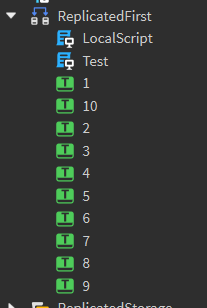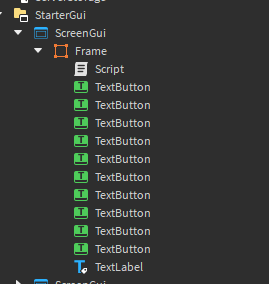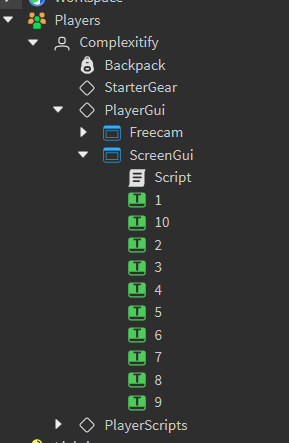# How to name each item a random number taken from a table

You can write your topic however you want, but you need to answer these questions:

1. What do you want to achieve? I want to be able to name each TextButton a number, ranging from 1-10 using tables.

2. What is the issue? I can’t seem to figure out how to create an efficient loop to do so.

3. What solutions have you tried so far? Looking on the devforum hasn’t helped for me and Youtube.

``````for i,v in pairs(script.Parent:GetChildren()) do
if v:IsA("TextButton") then -- Checks to see if the child is a textbutton
local numrandomtable = {1, 2, 3, 4, 5, 6, 7, 8, 9 ,10}
local picked = 0
for i = 1, 10 do
repeat
picked = numrandomtable[math.random(1, #numrandomtable)]
until not table.find(alreadyPicked, picked) -- Checks to see if the random number has been selected
v.Name = picked
end
end
end
``````
3 Likes

The code looks ok, but you could try this if you want efficiency:

``````local random_numbers = {1, 2, 3, 4, 5, 6, 7, 8, 9, 10}
-- If you want to call this loop multiple times, you will need to reset the random_numbers each time.

for i,v in pairs(script.Parent:GetChildren()) do
if v:IsA("TextButton") then -- Checks to see if the child is a textbutton
local picked = math.random(1, #random_numbers)
local number = random_numbers[picked]

v.Name = tostring(number)
table.remove(random_numbers, picked) -- Remove it from the array so it won't be picked again.
end
end
``````

How many TextButtons are there? Because the code above assumes 10.

Hope this helps!

1 Like

There are ten TextButtons total. I tried your code but it didn’t seem to work.

1 LikeThis is what I got when I ran it.

Can I see where the script is?

1 Like

I see that you put it in ReplicatedFirst as a local script. My intentions were to leave in a GUI as a serverscript but now I assume that it will only work as a local script. Is that the case?

1 Like

That shouldn’t matter as far as i’m concerned.

1 Like

Another method would be to shuffle the table and then just iterate in order:

``````-- https://stackoverflow.com/a/68486276
local function ShuffleInPlace(t)
for i = #t, 2, -1 do
local j = math.random(i)
t[i], t[j] = t[j], t[i]
end
end

local boxes = script.Parent:GetChildren()
ShuffleInPlace(boxes)
for i, v in boxes do
if v:IsA("TextBox") then
v.Name = tostring(i)
end
end
``````
1 Like

I used the exact same code using a server script and inside of a screengui but it still doesn’t work though.

1 LikeI apologize for being vague, but my intentions were to make this script run in a regular ScreenGui. Despite your code working in ReplicatedFirst as a LocalScript, it doesn’t work when I try it this way. Is there any way to make it so it runs as a server script in a gui?

You are looking under StarterGui, which doesn’t show the actual GUI on your screen.You need to look under PlayerGui inside your Player to find the ScreenGui.

Try looking under PlayerGui and tell me if it still doesn’t work.

Hope this helps!

1 Like

### My solution

``````-- What is the number of numbers?
local NUMBER_RANGE = 10

-- Used to store what numbers have already been used
local UsedNumbers = {}

-- Gets a number that has not yet been used
-- Warns "All numbers used, re-using a random one" if no unused ones exist
-- Will always return a number within the range
local function GetRandomUnusedNumber() : number
if #UsedNumbers >= NUMBER_RANGE then
-- All numbers were used, fall back

warn("All numbers used, re-using a random one")
return math.random(1, 10)
else
-- If there are unused numbers, pick a random one

local NumberOptions = {}
local PickedNumber

for Number = 1, NUMBER_RANGE do
if not table.find(UsedNumbers, tostring(Number)) then
-- If the number has not been used, add it to options to pick from

table.insert(NumberOptions, tostring(Number))
end
end

PickedNumber = NumberOptions[math.random(1, #NumberOptions)]
table.insert(UsedNumbers, tostring(PickedNumber))

return PickedNumber
end
end

-- Resets the data containing what numbers were use
local function ResetUsedNumbers()
UsedNumbers = {}
end

---------------------------------------------------------------------
---------------------------------------------------------------------
---------------------------------------------------------------------

-- Testing:

for _ = 1, 12 do
local Number = GetRandomUnusedNumber()
print(`Selected number: {Number}`)
end

ResetUsedNumbers()

for _ = 1, 5 do
local Number = GetRandomUnusedNumber()
print(`Selected number: {Number}`)
end
``````

### Sample output:

``````--[[

Selected number: 4  -  Server - Script:38
Selected number: 9  -  Server - Script:38
Selected number: 8  -  Server - Script:38
Selected number: 5  -  Server - Script:38
Selected number: 3  -  Server - Script:38
Selected number: 10  -  Server - Script:38
Selected number: 2  -  Server - Script:38
Selected number: 6  -  Server - Script:38
Selected number: 7  -  Server - Script:38
Selected number: 1  -  Server - Script:38
All numbers used, re-using a random one  -  Server - Script:9
Selected number: 6  -  Server - Script:38
All numbers used, re-using a random one  -  Server - Script:9
Selected number: 9  -  Server - Script:38
Selected number: 7  -  Server - Script:45
Selected number: 4  -  Server - Script:45
Selected number: 5  -  Server - Script:45
Selected number: 8  -  Server - Script:45
Selected number: 3  -  Server - Script:45

--]]
``````

Oh. It does work. Thanks for your solution! But how would you re-shuffle the names after?

I’m very sorry for the late reply.

Here is a function that you can use. It can be called as many times as needed.

``````local function NameRandomNumbers(items)
local random_numbers = {}

-- Add a number for each child.
for i = 1, #items do
random_numbers[i] = i
end

-- This loop shuffles the numbers and renames each child randomly.
for i,v in pairs(items) do
-- This only works for textbuttons, but you can change it by removing the if statement.
if (v:IsA("TextButton")) then -- Checks to see if the child is a textbutton
local picked = math.random(1, #random_numbers)
local number = random_numbers[picked]

v.Name = tostring(number)
table.remove(random_numbers, picked) -- Remove it from the array so it won't be picked again.
end
end
end

-- Example of how you might use it.
NameRandomNumbers(script.Parent:GetChildren())
``````

Hope this helps!

This topic was automatically closed 14 days after the last reply. New replies are no longer allowed.